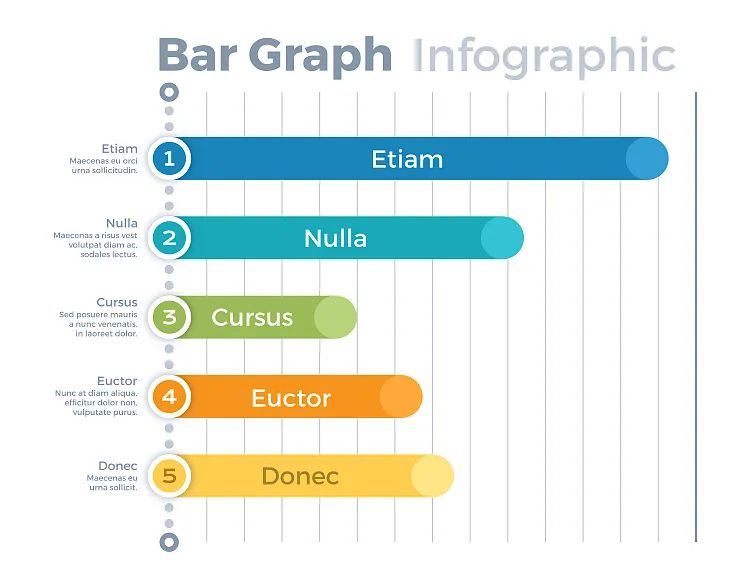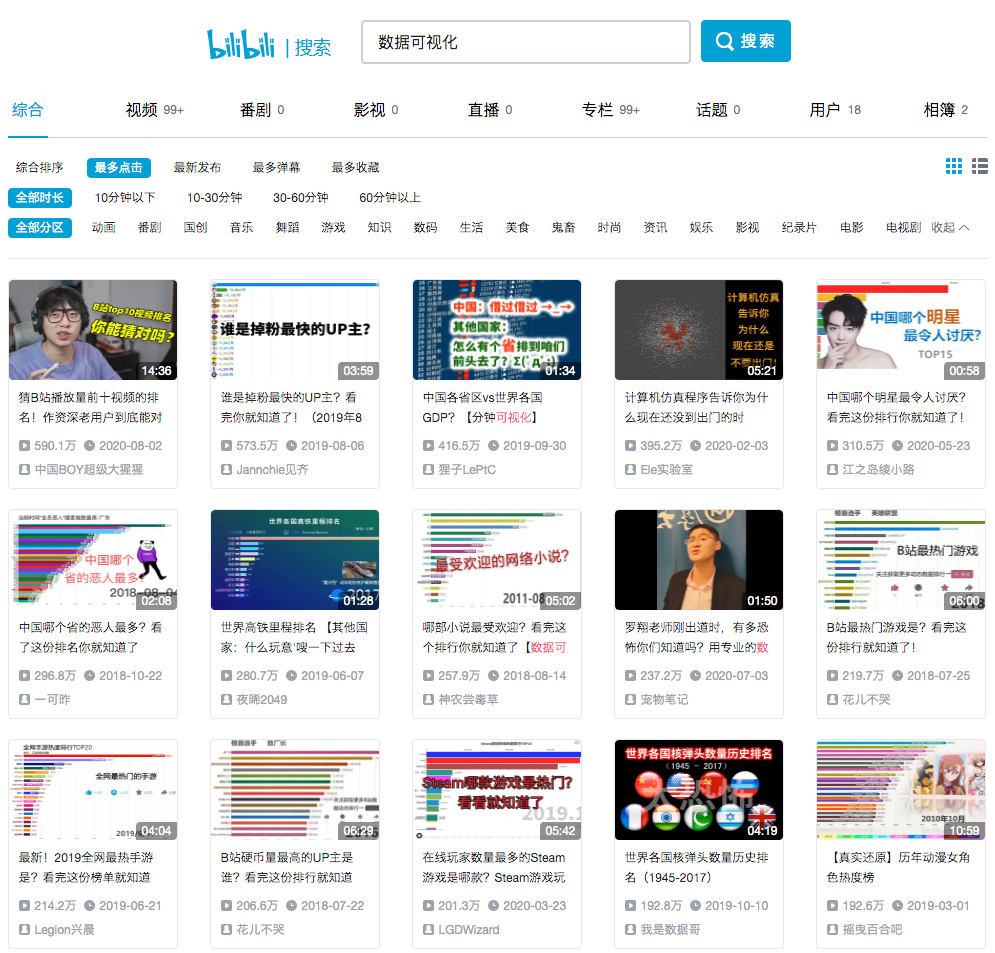GitHub地址：

https://github.com/dexplo/bar_chart_race

https://www.dexplo.org/bar_chart_race/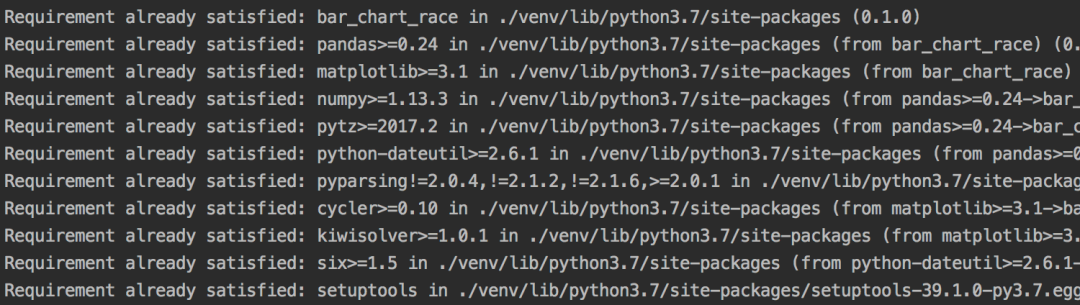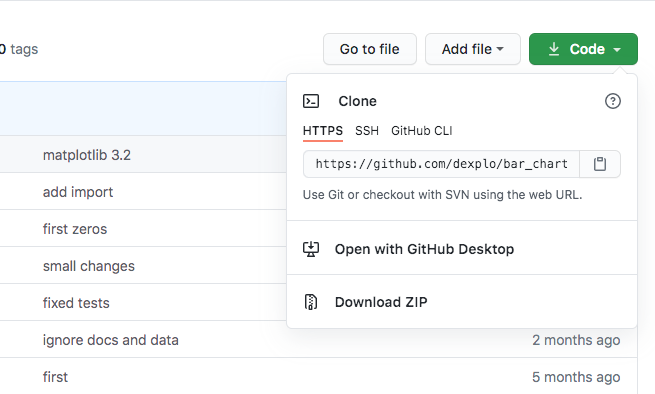``````cd 你的项目地址/venv/lib/python3.7/site-packages/bar_chart_race-master
python setup.py install

# 提示成功安装
# Finished processing dependencies for bar-chart-race==0.2.0
``````

``````import bar_chart_race as bcr

# 如果出现SSL错误,则全局取消证书验证
# import ssl
# ssl._create_default_https_context = ssl._create_unverified_context

# 获取数据
# print(df)

# 生成GIF图像
bcr.bar_chart_race(df, 'covid19_horiz.gif')
``````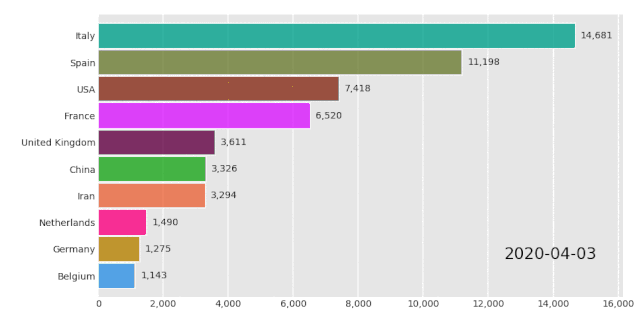3行代码Python代码就实现了，对大佬封装好的库表示膜拜～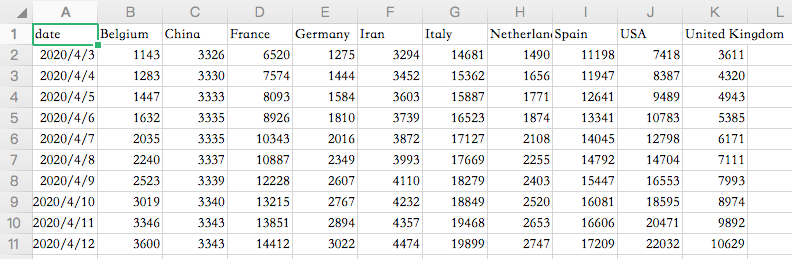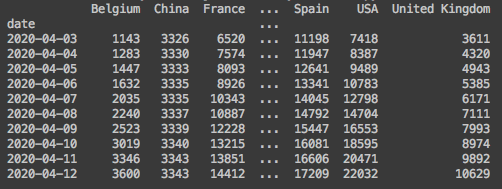1、动态条形图变动态柱状图

``````# orientation='v',生成柱状图
bcr.bar_chart_race(df, 'covid19_horiz.gif', orientation='v')
``````
``````

``````

2、排序方式，默认为降序(desc)

``````# 设置排序方式,asc-升序
bcr.bar_chart_race(df, 'covid19_horiz.gif', sort='asc')
``````3、条目数限制，此处设置为最多出现6条

``````# 设置最多能显示的条目数,6条
bcr.bar_chart_race(df, 'covid19_horiz.gif', n_bars=6)
``````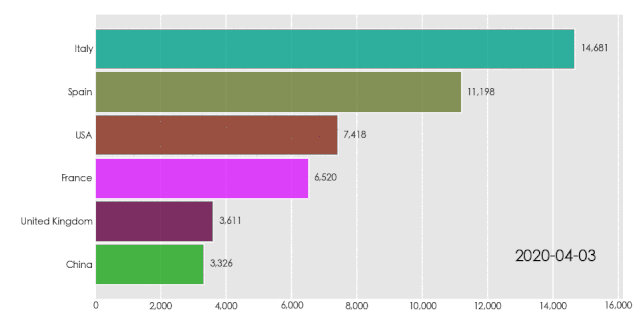4、设置固定类目

``````# 选取如下5个国家的数据
bcr.bar_chart_race(df, 'covid19_horiz.gif', fixed_order=['Iran', 'USA', 'Italy', 'Spain', 'Belgium'])
``````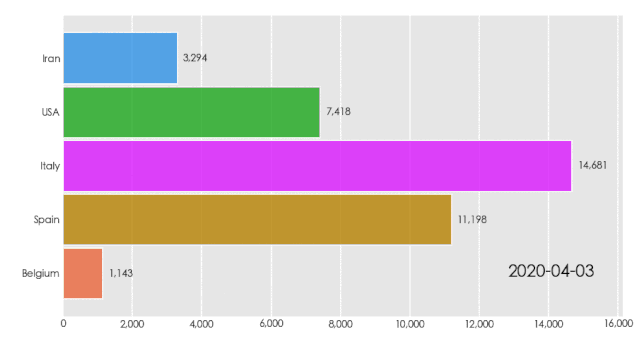5、固定数值轴，使其不发生动态变化

``````# 设置数值的最大值，固定数值轴bcr.bar_chart_race(df, 'covid19_horiz.gif', fixed_max=True)
``````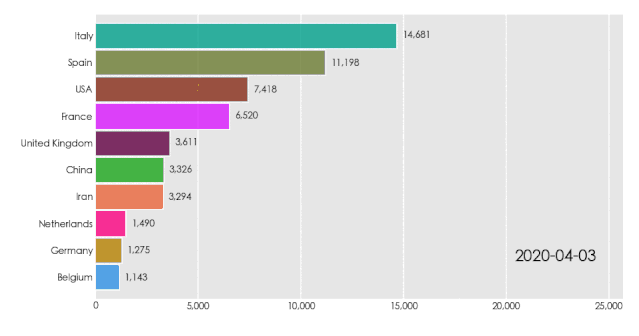6、图像帧数，默认10帧，此处设置为3帧，可以发现图像明显变得有些卡顿

``````# 图像帧数。数值越小，越不流畅。越大，越流畅。
bcr.bar_chart_race(df, 'covid19_horiz.gif', steps_per_period=3)
``````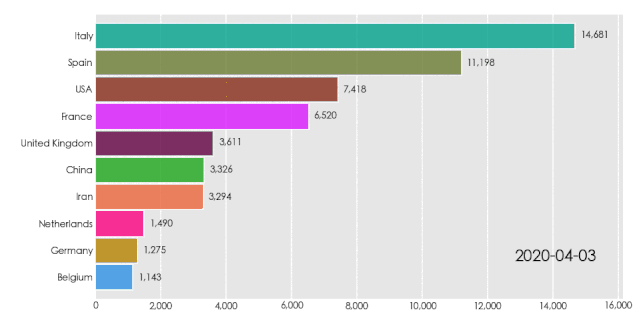7、设置帧率，单位时间默认为500ms

``````# 设置20帧的总时间，此处为200ms
bcr.bar_chart_race(df, 'covid19_horiz.gif', steps_per_period=20, period_length=200)
``````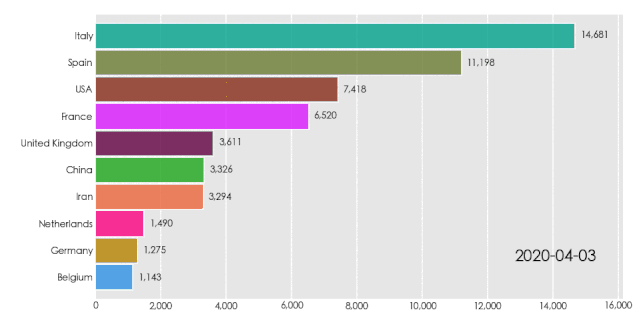8、设置每帧增加的标签时间，默认为False

``````# 输出MP4
bcr.bar_chart_race(df, 'covid19_horiz.mp4', interpolate_period=True)
``````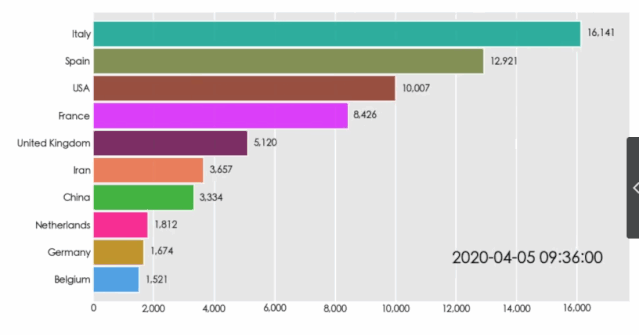9、绘图属性设置

``````# figsize-设置画布大小，默认(6, 3.5)
# dpi-图像分辨率，默认144
# label_bars-显示柱状图的数值信息，默认为True
# period_label-显示时间标签信息，默认为True
# title-图表标题
bcr.bar_chart_race(df, 'covid19_horiz.gif', figsize=(5, 3), dpi=100, label_bars=False,
period_label={'x': .99, 'y': .1, 'ha': 'right', 'color': 'red'},
title='COVID-19 Deaths by Country')
``````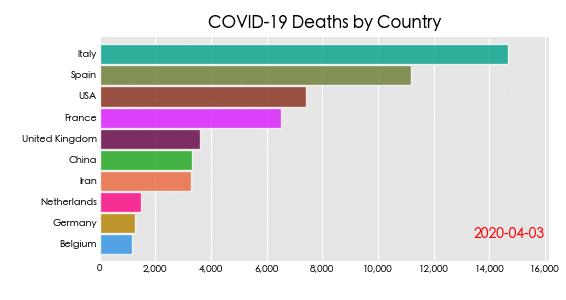10、配置标签文字大小

``````# bar_label_size-柱状图标签文字大小
# tick_label_size-坐标轴标签文字大小
# title_size-标题标签文字大小
bcr.bar_chart_race(df, 'covid19_horiz.gif', bar_label_size=4, tick_label_size=5,
title='COVID-19 Deaths by Country', title_size='smaller')
``````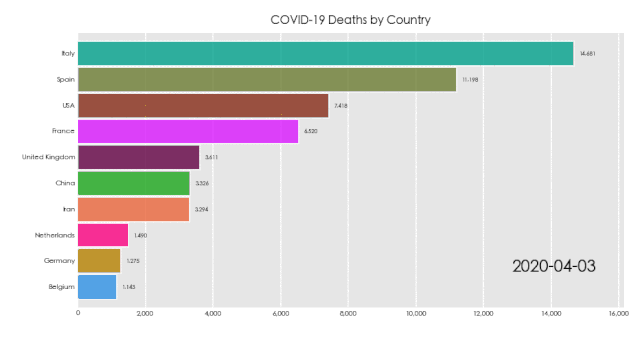11、全局文字属性

``````# shared_fontdict-全局字体属性
bcr.bar_chart_race(df, 'covid19_horiz.gipurple'})
``````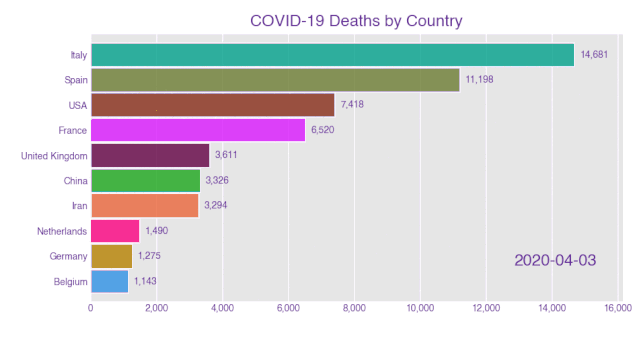12、条形图属性，可以设置透明度，边框等

``````# bar_kwargs-条形图属性
bcr.bar_chart_race(df, 'covid19_horiz.gif', bar_kwargs={'alpha': .2, 'ec': 'black', 'lw': 3})
``````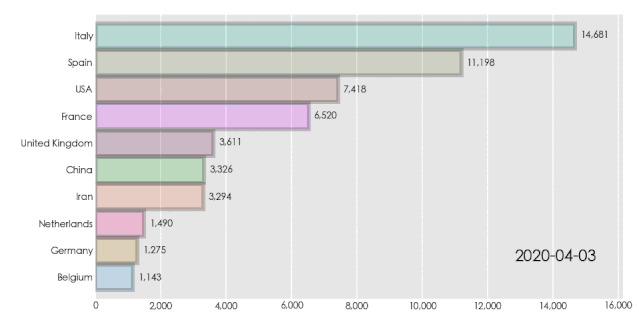13、设置日期标签的时间格式

``````# 设置日期格式，默认为'%Y-%m-%d'
bcr.bar_chart_race(df, 'covid19_horiz.gif', period_fmt='%b %-d, %Y')
``````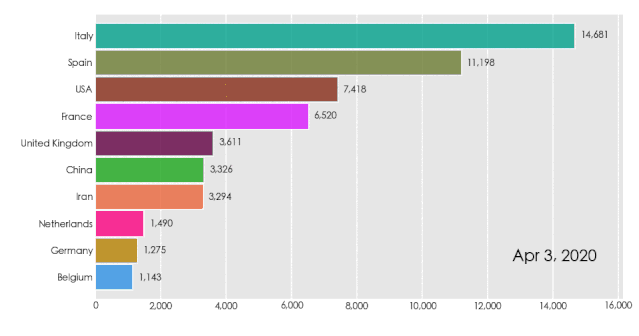14、更改日期标签为数值

``````# 设置日期标签为数值
bcr.bar_chart_race(df.reset_index(drop=True), 'covid19_horiz.gif', interpolate_period=True,
period_fmt='Index value - {x:.2f}')
``````
``````                                 period_fmt='Index value - {x:.2f}')

``````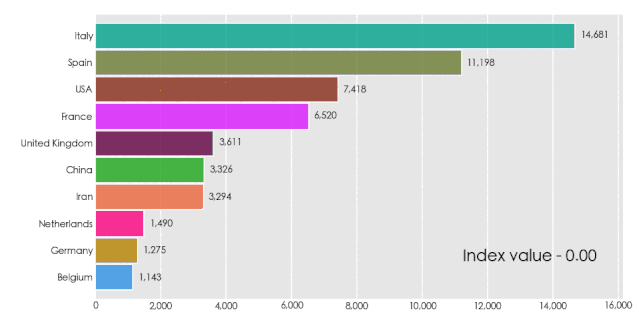15、添加动态文本，此处为数值总数统计

``````# 设置文本位置、数值、大小、颜色等
def summary(values, ranks):
total_deaths = int(round(values.sum(), -2))
s = f'Total Deaths - {total_deaths:,.0f}'
return {'x': .99, 'y': .05, 's': s, 'ha': 'right', 'size': 8}
# 添加文本
bcr.bar_chart_race(df, 'covid19_horiz.gif', period_summary_func=summary)
``````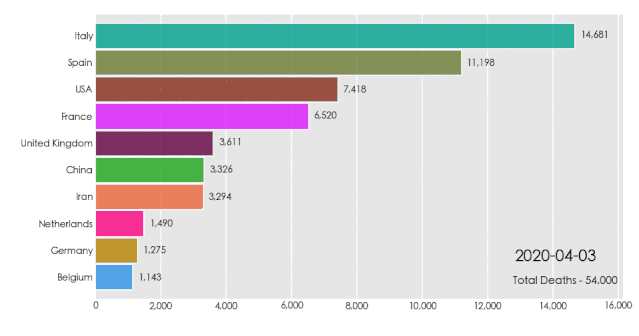16、添加垂直条，可选类型有平均值、分位数等

``````# 设置垂直条数值，分位数
def func(values, ranks):
return values.quantile(.9)
# 添加垂直条
bcr.bar_chart_race(df, 'covid19_horiz.gif', perpendicular_bar_func=func)
``````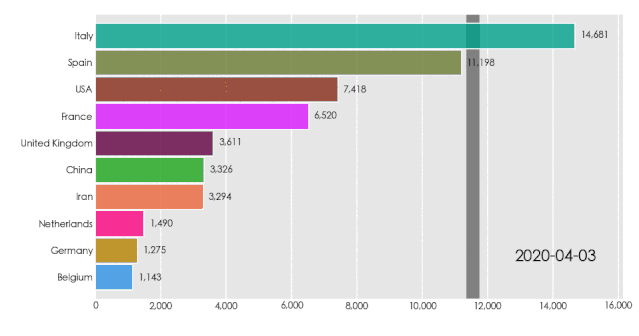17、设置柱状图颜色，默认为dark24

``````# 设置柱状图颜色
bcr.bar_chart_race(df, 'covid19_horiz.gif', cmap='accent')
``````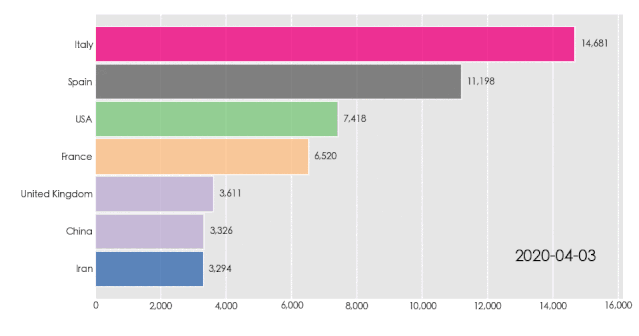18、柱状图颜色不重复，上面这个图是有重复颜色的

``````# 去除重复颜色
bcr.bar_chart_race(df, 'covid19_horiz.gif', cmap='accent', filter_column_colors=True)
``````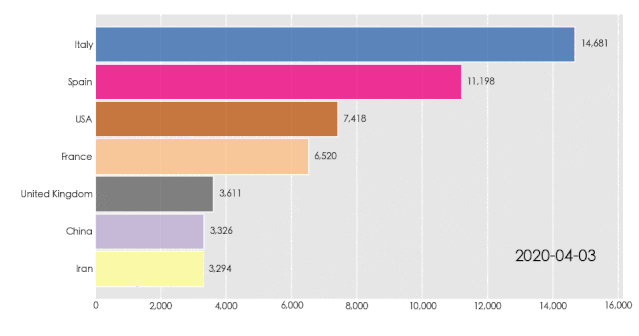``````#中文显示
plt.rcParams['font.sans-serif'] = ['SimHei']  #Windows
plt.rcParams['font.sans-serif'] = ['Hiragino Sans GB'] #Mac
plt.rcParams['axes.unicode_minus'] = False
``````

``````import bar_chart_race as bcr
import pandas as pd

# 读取数据
# 处理数据
df_result = pd.pivot_table(df, values='number', index=['day'], columns=['name'], fill_value=0)
# print(df_result)

# 生成图像
bcr.bar_chart_race(df_result, 'heat.gif', title='我是余欢水演职人员热度排行')
``````
``````

``````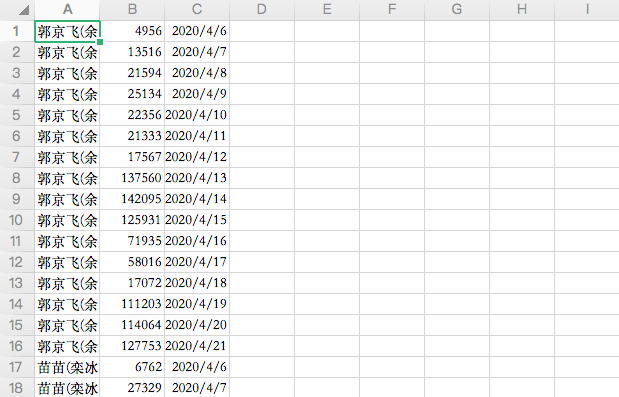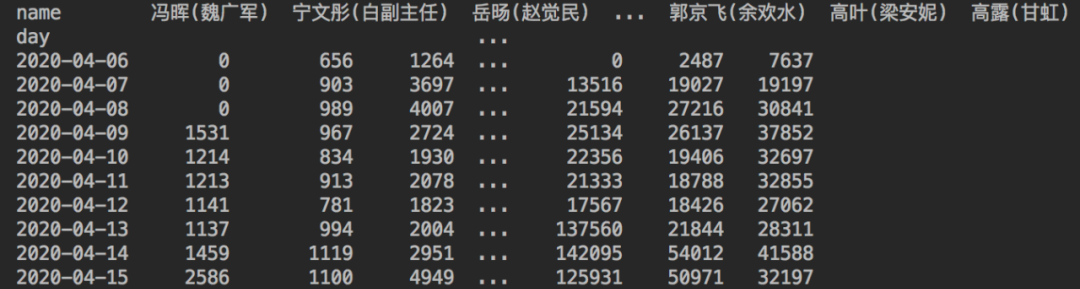``````colormaps =
{
"new_colors": [
'#ff812c',
'#ff5a5a',
'#00c5d2',
'#a64dff',
'#4e70f0',
'#f95dba',
'#ffce2b'
]
}
``````

``````# 使用自定义的颜色列表
bcr.bar_chart_race(df_result, 'heat.gif', title='我是余欢水演职人员热度排行', cmap='new_colors')
``````
``````

``````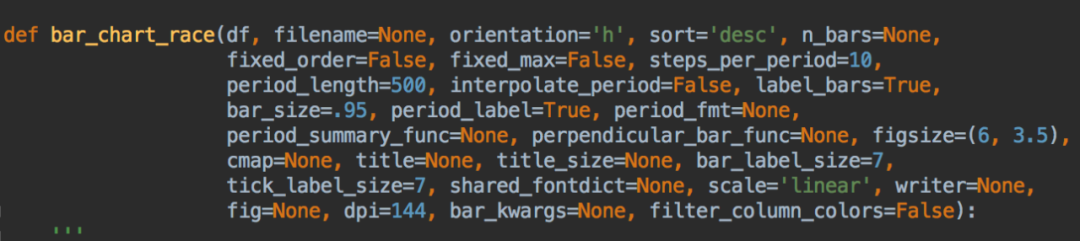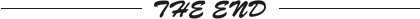``````更多精彩推荐
☞JavaScript 爆红后，微软为何还要开发 TypeScript？
☞李开复对话彭特兰：AI 不是单打独斗，应避免 AI 冷战！

☞如何应对云原生之旅中的安全挑战？
☞自拍卡通化，拯救动画师，StyleGAN再次玩出新花样
☞还不懂Redis？看完这个故事就明白了！
☞区块链+生鲜：杜绝“偷梁换柱”和“以次充好”
``````
``````点分享点点赞点在看
``````20年前，《新程序员》创刊时，我们的心愿是全面关注程序员成长，中国将拥有新一代世界级的程序员。20年后的今天，我们有了新的使命：助力中国IT技术人成长，成就一亿技术人！